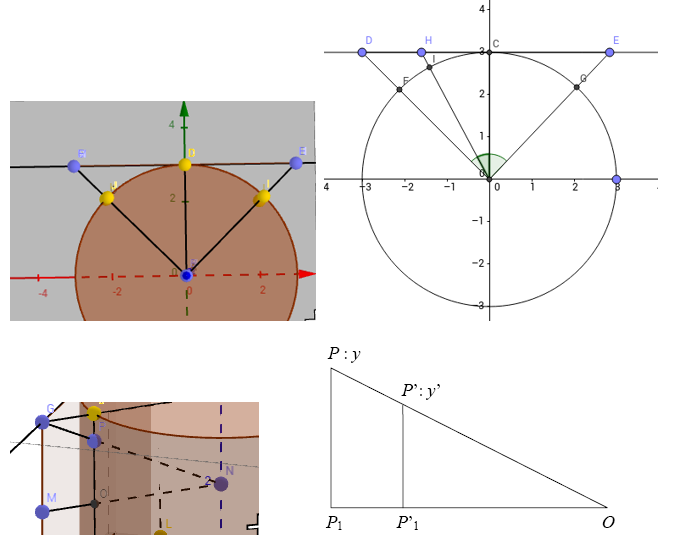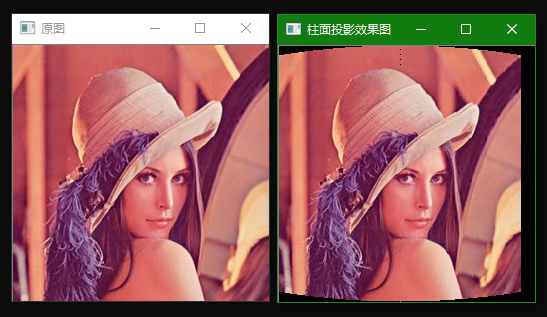2017-07-26 21:40:13 dongdong_csdn 阅读数 3511``````void cylinder()
{
int i,j;
double f;
int x,y;
double halfIMGROWS,halfIMGCOLS;
halfIMGROWS=IMGROWS/2;
halfIMGCOLS=IMGCOLS/2;
f=halfIMGCOLS/(tan(CYLINDERF/2));   //可调整参数CYLINDERF
for(i=0;i<IMGROWS;++i)
{
for(j=0;j<IMGCOLS;++j)
{
x=(int)((i-halfIMGROWS)*sqrt((j-halfIMGCOLS)*(j-halfIMGCOLS)+f*f)/f+halfIMGROWS+0.5);
y=(int)(f*tan((j-f*atan(halfIMGCOLS/f))/f)+halfIMGCOLS+0.5);
if(x<IMGROWS&&y<IMGCOLS)
{//彩色图像，BGR三个通道
cylinderMatrix1[i][j*3]=BMP1.mateix[x][y*3];
cylinderMatrix1[i][j*3+1]=BMP1.mateix[x][y*3+1];
cylinderMatrix1[i][j*3+2]=BMP1.mateix[x][y*3+2];
}
}
}
len=2*(f*atan(-halfIMGCOLS/f)+halfIMGCOLS);
//  printf("%f",len);
///////以下是拉伸///////////
for(i=0;i<IMGROWS;++i)
{
for(j=0;j<IMGCOLS;++j)
{
BMP1.mateix[i][j*3]=cylinderMatrix1[i][y];
BMP1.mateix[i][j*3+1]=cylinderMatrix1[i][y+1];
BMP1.mateix[i][j*3+2]=cylinderMatrix1[i][y+2];
}
}
}``````

2018-10-06 21:28:33 Young__Fan 阅读数 1154

## 原理:设原图像宽W，高H，角度FOG为相机视场角度α（一般为45°，即PI/4），圆形半径（焦距）f 有tan 1/2α = W / (2 * f), 则有f = W / (2 * tan(α/2))。

依次推算出，目标图像的宽（曲线FCG长）W‘ = f * α， 目标图像高H’不变， H‘ = H## 下面使用OpenCV进行代码实现

### 1.直接根据方式一进行代码实现

``````/**
*Copyright (c) 2018 Young Fan.All Right Reserved.
*Author: Young Fan
*Date: 2018.9.30
*OpenCV version: 3.4.3
*IDE: Visual Studio 2017
*Description:
*/

#include <iostream>
#include "opencv2/highgui/highgui.hpp"

using namespace cv;
using namespace std;

int main()
{
Mat dstImage = Mat::zeros(srcImage.size(), CV_8UC3);

int height = srcImage.rows; //原图像的高（即原图像矩阵行数）
int width = srcImage.cols; //原图像的宽（即原图像矩阵列数）
int centerX = width / 2; //图像中心横坐标
int centerY = height / 2; //图像中心纵坐标
double alpha = CV_PI / 4; //相机视角角度
double f = width / (2 * tan(alpha / 2)); //焦距（圆的半径）

//循环遍历
for (int i = 0; i < srcImage.rows; i++)
{
for (int j = 0; j < srcImage.cols; j++)
{
//注意图像坐标与像素矩阵坐标的区别
float theta = atan((j - centerX) / f);
int pointX = f * alpha / 2 + f * theta; //用f * alpha / 2,两边缝隙会不均匀（只有右边有黑缝隙），用width / 2就均匀了
int pointY = f * (i - centerY) / sqrt((j - centerX) * (j - centerX) + f * f) + centerY;

//像素赋值
dstImage.at<Vec3b>(pointY, pointX) = srcImage.at<Vec3b>(i, j);
dstImage.at<Vec3b>(pointY, pointX) = srcImage.at<Vec3b>(i, j);
dstImage.at<Vec3b>(pointY, pointX) = srcImage.at<Vec3b>(i, j);
}
}

imshow("原图", srcImage);
imshow("柱面投影效果图", dstImage);

waitKey();

return 0;
}``````

因为一张平面图像在进行柱面投影的时候，原图被转化为柱面图的形式显示在与原来尺寸一样的黑色背景图上，柱面投影效果图上有一部分像素点无法找到与原图与之对应坐标变换的点，所以会出现默认颜色为黑色的缝隙。按照直观的说法就是，原图转为柱面图像时在平面上被缩小了一些。

那么为啥，只有右边有缝隙，而左边没有呢？

这是因为推算的坐标变换公式所求出的柱面投影坐标，是在柱面上建立的坐标系，但是我们在代码实现并显示的时候，用的是一张平面图来呈现这个柱面图，也就是侧视图，所以要想得到左右对称图像，要在代码35行求横坐标的时候，将图像宽度要以原图的宽度为准，这样在显示的时候可以使左右缝隙更加对称。### 2.改进代码，是左右缝隙对称

``````/**
*Copyright (c) 2018 Young Fan.All Right Reserved.
*Author: Young Fan
*Date: 2018.9.30
*OpenCV version: 3.4.3
*IDE: Visual Studio 2017
*Description:
*/

#include <iostream>
#include "opencv2/highgui/highgui.hpp"

using namespace cv;
using namespace std;

int main()
{
Mat dstImage = Mat::zeros(srcImage.size(), CV_8UC3);

int height = srcImage.rows; //原图像的高（即原图像矩阵行数）
int width = srcImage.cols; //原图像的宽（即原图像矩阵列数）
int centerX = width / 2; //图像中心横坐标
int centerY = height / 2; //图像中心纵坐标
double alpha = CV_PI / 4; //相机视角角度
double f = width / (2 * tan(alpha / 2)); //焦距（圆的半径）

//循环遍历
for (int i = 0; i < srcImage.rows; i++)
{
for (int j = 0; j < srcImage.cols; j++)
{
//注意图像坐标与像素矩阵坐标的区别
float theta = atan((j - centerX) / f);
int pointX = width / 2 + f * theta; //注意这里用width / 2，用f * alpha两边缝隙不均匀（只有右边有黑缝隙）
int pointY = f * (i - centerY) / sqrt((j - centerX) * (j - centerX) + f * f) + centerY;

//像素赋值
dstImage.at<Vec3b>(pointY, pointX) = srcImage.at<Vec3b>(i, j);
dstImage.at<Vec3b>(pointY, pointX) = srcImage.at<Vec3b>(i, j);
dstImage.at<Vec3b>(pointY, pointX) = srcImage.at<Vec3b>(i, j);
}
}

imshow("原图", srcImage);
imshow("柱面投影效果图", dstImage);

waitKey();

return 0;
}``````### 3.如果你不想要出现黑色缝隙，可以把左右黑色缝隙去除

需要定义合适尺寸的目标图，然后就是目标图的列数（图像的横坐标）要左移，具体见下面代码。

``````/**
*Copyright (c) 2018 Young Fan.All Right Reserved.
*Author: Young Fan
*Date: 2018.10.6
*OpenCV version: 3.4.3
*IDE: Visual Studio 2017
*Description:
*/

#include <iostream>
#include "opencv2/highgui/highgui.hpp"

using namespace cv;
using namespace std;

int main()
{

int height = srcImage.rows; //原图像的高（即原图像矩阵行数）
int width = srcImage.cols; //原图像的宽（即原图像矩阵列数）
int centerX = width / 2; //图像中心横坐标
int centerY = height / 2; //图像中心纵坐标
double alpha = CV_PI / 4; //相机视角角度
double f = width / (2 * tan(alpha / 2)); //焦距（圆的半径）

//求左右黑色缝隙宽度
int len = cvRound(width / 2 - f * alpha / 2); //cvRound:取整

//定义合适的目标图
Mat dstImage = Mat::zeros(srcImage.rows, width - 2 * len, CV_8UC3); //注意尺寸

//循环遍历
for (int i = 0; i < srcImage.rows; i++)
{
for (int j = 0; j < srcImage.cols; j++)
{
//注意图像坐标与像素矩阵坐标的区别
float theta = atan((j - centerX) / f);
int pointX = cvRound(width / 2 + f * theta); //注意这里用width / 2，用f * alpha / 2,两边缝隙会不均匀（只有右边有黑缝隙）
int pointY = cvRound(f * (i - centerY) / sqrt((j - centerX) * (j - centerX) + f * f) + centerY);

//像素赋值,此时要将列数（图像横坐标）往左移，与初始的黑色图像边缘对其，即pointX - len
dstImage.at<Vec3b>(pointY, pointX - len) = srcImage.at<Vec3b>(i, j);
dstImage.at<Vec3b>(pointY, pointX - len) = srcImage.at<Vec3b>(i, j);
dstImage.at<Vec3b>(pointY, pointX - len) = srcImage.at<Vec3b>(i, j);
}
}

imshow("原图", srcImage);
imshow("柱面投影效果图", dstImage);

waitKey();

return 0;
}``````https://www.cnblogs.com/cheermyang/p/5431170.html

https://blog.csdn.net/wd1603926823/article/details/49334229

2019-04-30 17:59:57 tech_otaku0512 阅读数 248

（信息A：相机焦距+目标距离）
（信息B：图像变换尺度s，与相机焦距f成正比）
（信息C：相机水平方向的视角，即相机到源图像两边延伸点的射线之间的夹角）

x < W / 2, y < H / 2.
x < W / 2, y > H / 2.
x > W / 2, y < H / 2.
x > W / 2, y > H / 2.

x1 = (L / 2) - s * arctan((W / 2 - x0) / s) , x0 <= (W / 2)
x1 = (L / 2) + s * arctan((x0 - W / 2) / s) , (W / 2) < x0 <= W

y1 = (H / 2) - s * (H / 2 - y0) /√ ((W / 2 - x0）^ 2 + s ^ 2) , y0 <= (H / 2)
y1 = (H / 2) + s * (y0 - H / 2) /√ ((W / 2 - x0）^ 2 + s ^ 2) , (H / 2) < y0 <= H

``````#include "pch.h"
#include <iostream>
#include <opencv2/opencv.hpp>
#include "opencv2/highgui/highgui.hpp"
#include "opencv2/imgproc/imgproc.hpp"

#define PI 3.141592653

using namespace std;
using namespace cv;

int main()
{
Mat img0, img1;
//img1 = img0.clone();
//img1.setTo(Scalar::all(0));
int W = img0.cols;							//W为图像的平面宽度
int H = img0.rows;							//H为图像的柱面高度
float L;									//目标柱面的宽度
float a = PI / 6;							//已知相机能看到的半角大小
float s = W / 2 / (tan(a));					//s为相机的焦距
int x0, y0;									//图像平面上某一点的横纵坐标（x0, y0）
float x1, y1;								//目标曲面上对应点的横纵坐标（x1, y1）
int zsx, zsy;								//整数x1和y1

L = 2 * s * a;								//求解L的总长度

int springX = (int)L * tan(a) / a +1;
img1.create(cvSize((int)L,H), img0.type());
img1.setTo(Scalar::all(0));
//cout << img0.size() << endl;
//cout << springX << endl;

double t0 = (double)getTickCount();
//分类讨论x0<=(W/2)和(W/2)<x0<=(W)以及y0<=(H/2)和(H/2)<y0<=(H)的情况，之后指令向量化优化
for (y0 = 1; y0 <= H; y0++)
{
for (x0 = 1; x0 <= W; x0++)
{
if (x0 <= (W / 2))
x1 = (L / 2) - s * cvFastArctan((float)(W / 2 - x0), s) * PI / 180;
else
if (x0 > (W / 2))
x1 = (L / 2) + s *cvFastArctan((float)(x0 - (W / 2)), s) * PI / 180;
if (y0 <= (H / 2))
y1 = (H / 2) - s * (H / 2 - y0) / sqrt(pow((W / 2) - x0, 2) + pow(s, 2));
else
if (y0 > (H / 2))
y1 = (H / 2) + s * (y0 - H / 2) / sqrt(pow((W / 2) - x0, 2) + pow(s, 2));

//化为整数坐标
zsx = (int)(x1);
zsy = (int)(y1);

//cout << L << endl;
//cout << zsx << endl;

if (zsx < W && zsy < H && x0<W && y0<H)
{
//cout << zsy << endl;
//cout << zsx << endl;
//cout << y0 << endl;
//cout << x0 << endl;
img1.at<Vec3b>(zsy, zsx) = img0.at<Vec3b>(y0, x0);
img1.at<Vec3b>(zsy, zsx) = img0.at<Vec3b>(y0, x0);
img1.at<Vec3b>(zsy, zsx) = img0.at<Vec3b>(y0, x0);
}
}
}
double t_proc = ((double)getTickCount() - t0) / getTickFrequency();
cout << "柱面投影：" << t_proc << endl;
cout << "变换尺度：" << s << endl;

cout << "柱面变换结束..." << endl;
imshow("cylindralCathedrale.jpg", img1);
imwrite("cylindralCathedrale.jpg", img1);
waitKey();

/*		向img1赋值
B0 = *(img0.data + img0.step * y0 + img0.step * x0 + img0.elemSize1() * 0);
*(img1.data + img1.step * zsy + img1.step * zsx + img1.elemSize1() * 0) = B0;
G0 = *(img0.data + img0.step * y0 + img0.step * x0 + img0.elemSize1() * 1);
*(img1.data + img1.step * zsy + img1.step * zsx + img1.elemSize1() * 1) = G0;
R0 = *(img0.data + img0.step * y0 + img0.step * x0 + img0.elemSize1() * 2);
*(img1.data + img1.step * zsy + img1.step * zsx + img1.elemSize1() * 2) = R0;
*/

/*
//测试cvFastArctan(分子, 分母),可用，得到的是角度制需要乘以PI/180转化为弧度制算弧长;
float testsum;
float testitem = 1.7320508075689 * W;
testsum = cvFastArctan(W, testitem);
cout << "结果在这里： " << endl;
cout << testsum << endl;
*/
}

``````

https://blog.csdn.net/czl389/article/details/54599253

https://www.cnblogs.com/cheermyang/p/5431170.html

2016-02-02 02:53:21 oHanTanYanYing 阅读数 10209

图像的柱面投影算法，在360°环形全景应用中几乎一定会用到。而为何要用该算法，可以参考下图:从图像中可以看到，該环形全景设备由八个摄像头环形排列而成（需注意环形全景的形态并不固定，摄像头的个数不一定是八个，甚至只有一个摄像头在一直匀速转圈也是可以的）。每个摄像头所拍摄的画面为其前方的实线段区域，为了之后能进行图像的拼接，相邻摄像头之间必须要有图像的重合区域，如上图的红色线段部分（如果能保证刚刚好相接也可以，不过结构难度太高）。

从不同摄像头的重合区域可以看到，由于摄像头的朝向不同，重合部分图像中的物体并不满足视觉一致性的要求，因此需要将图像进行投影，使其满足图像的一致性要求，为后面的拼接做准备（视觉一致性是全景应用最为关键的问题，无论是柱环形全景还是球形全景，都无法避免，只是所选的投影模型不同罢了）。在环形全景中，一般选择柱面投影算法，将图像分别投影到以 像素焦距+摄像头与圆心距离 为半径的圆柱上。投影后的图像为上图摄像头前方的圆弧。从圆弧上看，图像的重合部分已经满足视觉一致性的要求，可以做拼接。而如何去投影就是本文要介绍的。

柱面投影的数学模型相对比较简单，把观测点定在圆柱体的中心，图像的像素焦距+摄像头与圆心距离 为圆柱体的半径，则摄像头所拍摄的图像与圆柱体相切。图像上的一点Q与观测点连线，该连线与圆柱面的交点点Q'为图像点Q在柱面上的投影，我们需要做的就是求出点Q(x,y)与点Q'(x',y')之间的换算关系。先看看下图:该图的实线部分为投影模型的俯视图，下方的线段a为待投影的图像，圆为圆柱切面，O为观测点。而虚线部分为Y轴方向上的辅助线。现在设A为图像上的任意一点，其坐标为(x,y,z),其中z=-R，则其在x-z坐标系上的投影A'的坐标为(x，-R)。B为我们要求的点A在圆柱面上的投影点，其坐标为(x',y',z')，则其在x-z坐标系上的投影点B'的坐标为(x',z')。

由于△0BB'与△OAA'相似，△0B'F与△OA'G相似

则有BB'=kAA',B'F=kA'G，OF=kR(k<1)

则kx=x',ky=y'

又OF²+B'F²=R²

故k²R²+k²x²=R²

又x'=kx,y'=ky

故

由于一般来说图像以左上角为坐标原点，而上面公式中的坐标系以图像的中心为坐标原点，所以在实际图像的计算中，上面的计算公式换为

有了上面的公式，便可计算出图像的柱面投影结果。这里需要注意的是我们把x和y写在了等式的左边而x',y'写在了右边，这样做是为了方便我们后面进行插值计算。为什么要这么做可以看下面两张图片左边图像为待投影图像，右边为直接投影的结果，由于投影后的图像点坐标未必为整数，而图像的坐标需要为整数，所以必将造成误差。表现在右边图像上就是图像有很多显而易见的毛刺。而我们进行双线性插值之后的投影图像如下可以看到，其毛刺得到了一定的抑制。上面的图像还说明了经过柱面投影的图像会比原来的图像宽度小，具体小多少跟R有关，由于比较简单，在这里不再给出推导的过程。以上投影过程的主要代码如下

```void DealWithImgData(BYTE *srcdata, BYTE *drcdata,int width,int height)//参数一为原图像的数据区首指针，参数二为投影后图像的数据区首指针，参数三为图像的宽，参数四为图像的高
{
//双线性插值算法
int i_original_img_hnum, i_original_img_wnum;//目标点坐标
double distance_to_a_y, distance_to_a_x;//在原图像中与a点的水平距离
int original_point_a, original_point_b, original_point_c, original_point_d;

int l_width = WIDTHBYTES(width* 24);//计算位图的实际宽度并确保它为4byte的倍数
int drcpoint;
double R = 1200;//像素距离
double x, y;
for (int hnum = 0; hnum < height; hnum++)
{
for (int wnum = 0; wnum < width; wnum++)
{
drcpoint = l_width*hnum + wnum * 3;//数组位置偏移量，对应于图像的各像素点RGB的起点
//柱面投影
double k = R / sqrt(R*R + (wnum- width / 2) * (wnum - width / 2));
x = (wnum - width / 2) / k + width / 2;
y = (hnum - height / 2) / k + height / 2;
if (x >= 0 && y >= 0 && x < width && y < height)
{
/***********双线性插值算法***********/
i_original_img_hnum = y;
i_original_img_wnum = x;
distance_to_a_y = y - i_original_img_hnum;
distance_to_a_x = x - i_original_img_wnum;//在原图像中与a点的垂直距离

original_point_a = i_original_img_hnum*l_width + i_original_img_wnum * 3;//数组位置偏移量，对应于图像的各像素点RGB的起点,相当于点A
original_point_b = original_point_a + 3;//数组位置偏移量，对应于图像的各像素点RGB的起点,相当于点B
original_point_c = original_point_a + l_width;//数组位置偏移量，对应于图像的各像素点RGB的起点,相当于点C
original_point_d = original_point_c + 3;//数组位置偏移量，对应于图像的各像素点RGB的起点,相当于点D

if (i_original_img_hnum == height - 1)
{
original_point_c = original_point_a;
original_point_d = original_point_b;
}
if (i_original_img_wnum == width - 1)
{
original_point_a = original_point_b;
original_point_c = original_point_d;
}

drcdata[drcpoint + 0] =
srcdata[original_point_a + 0] * (1 - distance_to_a_x)*(1 - distance_to_a_y) +
srcdata[original_point_b + 0] * distance_to_a_x*(1 - distance_to_a_y) +
srcdata[original_point_c + 0] * distance_to_a_y*(1 - distance_to_a_x) +
srcdata[original_point_c + 0] * distance_to_a_y*distance_to_a_x;
drcdata[drcpoint + 1] =
srcdata[original_point_a + 1] * (1 - distance_to_a_x)*(1 - distance_to_a_y) +
srcdata[original_point_b + 1] * distance_to_a_x*(1 - distance_to_a_y) +
srcdata[original_point_c + 1] * distance_to_a_y*(1 - distance_to_a_x) +
srcdata[original_point_c + 1] * distance_to_a_y*distance_to_a_x;
drcdata[drcpoint + 2] =
srcdata[original_point_a + 2] * (1 - distance_to_a_x)*(1 - distance_to_a_y) +
srcdata[original_point_b + 2] * distance_to_a_x*(1 - distance_to_a_y) +
srcdata[original_point_c + 2] * distance_to_a_y*(1 - distance_to_a_x) +
srcdata[original_point_c + 2] * distance_to_a_y*distance_to_a_x;
/***********双线性插值算法***********/
}
}
}
}```

2019-07-22 16:23:28 qq_39642978 阅读数 42